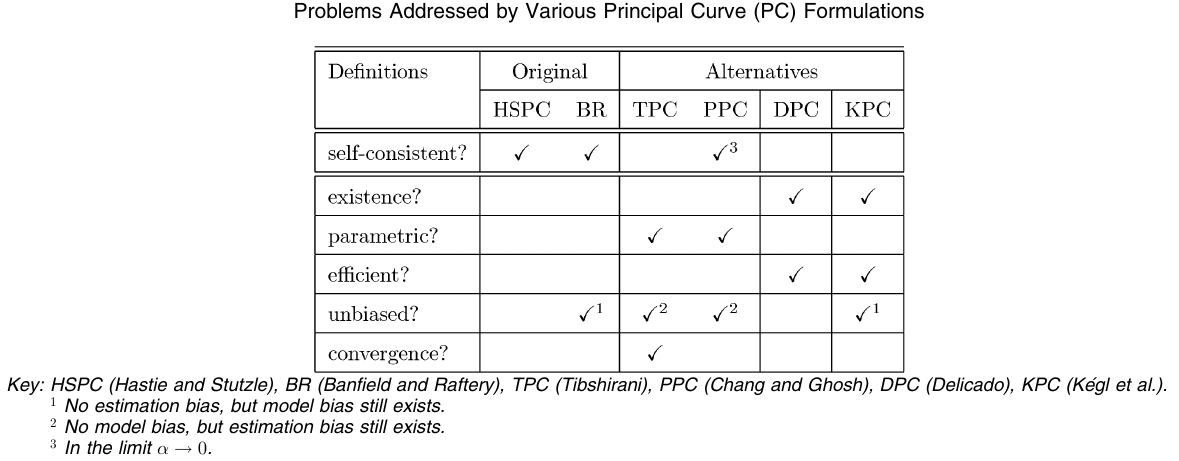# Probabilistic Principal Curves

##### Posted on Nov 22, 2021
Tags: Principal Curves

This note is for Chang, K.-Y., & Ghosh, J. (2001). A unified model for probabilistic principal surfaces. IEEE Transactions on Pattern Analysis and Machine Intelligence, 23(1), 22–41., but only involves the principal curves.

principal curves and surfaces are nonlinear generelizations of principal components and subspaces, respectively.

Solutions to several problems, such as proof of existence and convergence, faced by the original principal curve formulation have been proposed. But these solutions are not generally extensible to principal surfaces, the mere computation of which presents a formidable obstacle.

The paper proposed the probabilistic principal surface (PPS) to address a number of issues associated with current principal surface algorithms. PPS uses a manifold oriented covariance noise model, based on the generative topographical mapping (GTM), which can be viewed as a parametric formulation of Kohonen’s self-organizing map.

Building on the PPS, the paper introduces a unified covariance model that implements PPS $(0 < \alpha < 1)$, GTM $(\alpha=1)$, and the manifold-aligned GTM $(\alpha >1)$ by varying the clamping parameter $\alpha$.

The problems of the original principal surface formulation:

1. existence cannot be guaranteed for arbitrary distributions
2. theoretical analysis is not as straightforward as with parametric models due to its nonparametric formulation
3. inefficient for large sample size as all data points are needed to define a principal curve in practice
4. biased at points of large curvature
5. convergence of the corresponding estimation algorithm cannot be guaranteed.

They proposed two estimated algorithms, probabilistic principal curve (PPC), and probabilistic principal surface (PPS), to address problems 2-4.

• PPC estimates a principal curve with a cubic-spline smoothed mixture of oriented Gaussians
• PPS incorporates oriented Gaussians into the generative topographical mapping (GTM) framework to approximate a principal surface.

## Principal Curves

• HSPC: original formulation
• BR: follows HSPC definition, but estimate the error residuals instead of the actual curve, thereby reducing the estimation bias. However, it introduces numerical instabilities which may lead to a smooth but incorrect principal curve in practice.
• TPC: use a cubic-spline smoothed mixture of Gaussians
• PPC: a modified version of TPC, approximate the self-consistency by using oriented Gaussians in the mixture model whose variance along the tangential direction is attenuated by a factor $\alpha < 1$.
• KPC: define the PC as a finite-length curve that minimizes the MSE over all curves of equal or shorter length

Most problems associated with principal curves have been successfully addressed by redefining the PCHowever, none of the newer definitions are easily extensible to principal manifolds.

Published in categories Note